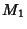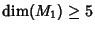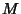## h-Cobordism Theorem

Ifis a Simply Connected, Compact Manifold with a boundary that has two components,and, such that inclusion of each is a Homotopy equivalence, thenis Diffeomorphic to the productfor. In other words, ifandare two simply connected Manifolds of Dimensionand there exists an h-Cobordismbetween them, thenis a productandis Diffeomorphic to.

The proof of the-cobordism theorem can be accomplished using Surgery. A particular case of the-cobordism theorem is the Poincaré Conjecture in dimension. Smale proved this theorem in 1961.

Smale, S. Generalized Poincaré's Conjecture in Dimensions Greater than Four.'' Ann. Math. 74, 391-406, 1961.